﻿ The Difference of Mathematical Problem Solving Ability through the Scientific Approach and the Scientific Approach Assisted by Software AutographPublications are Open
Access in this journal
Article Versions
Export Article
• Normal Style
• MLA Style
• APA Style
• Chicago Style
Research Article
Open Access Peer-reviewed

### The Difference of Mathematical Problem Solving Ability through the Scientific Approach and the Scientific Approach Assisted by Software Autograph

Rismalyah Manalu , E.Elvis Napitupulu, Martua Manullang, Delima Simanjuntak, Jetti H. Sinambela
American Journal of Educational Research. 2018, 6(12), 1693-1701. DOI: 10.12691/education-6-12-16
Received November 12, 2018; Revised December 14, 2018; Accepted December 27, 2018

### Abstract

The objectives of this study are: (1) Analyzing the presence or absence of differences in mathematical solving abilities between students taught using the scientific approach and the scientific approach assisted by software Autograph, (2) Testing statistically whether or not there is interaction between learning approaches (scientific, scientific assisted Autograph software) and mathematical initial ability (high, medium, low) to mathematical problem solving abilities. The study population is all students of class X Public Senior High School 1 Medan with a total of 464 people who have been distributed into 13 parallel classes, while the research sample is class X students of Mathematics and Natural Sciences-5 as Experiment-I Class and class X Mathematics and Natural Sciences- 6 as Experimental Class-II as many as 68 people. The instrument used is a test of mathematical problem solving abilities. Hypothesis testing uses two-way variant analysis techniques with 2x3 factorial design. In this study using two factors, namely: 1) The learning approach consists of two levels (scientific, scientific assisted software Autograph) and 2) initial mathematical abilities consisting of three levels (High, Medium and Low). The results of the study show: (1) there are significant differences in mathematical problem solving abilities between students taught using a scientific approach and a scientific approach assisted by software Autograph with a difference of = 0.0704, so the use of software Autograph contributes to improving students' mathematical problem solving abilities in the material of equations and inequalities of absolute linear values of one variable. (2) There is no interaction between the learning approach (scientific, scientific assisted Autograph software) and the initial ability (high, medium, low) on students' mathematical problem-solving abilities, so that the effect of the second factor of learning contributes to improving mathematical problem solving skills students and students' initial mathematical abilities only have a very small effect on improving problem solving abilities.

### 1. Introduction

Problem solving is one essential thing in mathematic school learning because (1) student become skilled in selecting, analyzing and examining relevant information (2) raises intellectual satisfaction from student internal (3) student intellectual potential increased (4) trains student in solving problem. Napitupulu stated “problem solving is undoubtedly the heart of mathematic and mathematic learning activities” 1. Hassoubah (Sofiyah) stated “problem solving is direction thinking to find a solution to way out of specific problem” 2. According to mathematicians incorporated with National Council of Teacher of Mathematics (NCTM) stated that “problem solving means engaging in a task for which the solution method is known in advance” 3. Based on experts opinion above concluded that problem solving is process to solve problem uses rules, combine mathematical concepts learned before in planning problem solving solution and solve problem solving questions based on predefined problem solving indicators.

Polya describes the process of problem solving at four stages, understanding the problem, determining the strategy, implementing the selected strategy and assessment 4. At the stage of understanding the problem, the students is expected to state what he understood from the problem and to determine what are the given and unknown in the problem and also to suggest clearly the condition of the problem. Charles, Lester & O’Daffer (Szetela and Nicol) arranged the indicator of each aspect or level of problem solving namely “(1) understanding problem (2) solving the problem and (3) answer the problem” 5. Schoenfeld declared that were numerous unaddressed issues dealing with problem solving instruction and assessment. Student are encouraged after problem solving process because problem solving contributes to the use of different solution and strategies development that students use 6.

The Third International Mathematics and Science Study (TIMSS) and Program for International Assessment of Student (PISA) diagnose result in 2015, Rahmawati expressed “student of Indonesia are weak in all aspect, content or cognitive either in Math or Science generally. Students of Indonesia master routine exercises, simple computation and measure knowledge based on facts to the daily context of students. Indonesia needs to strengthen the ability of integrating information, making conclusion and generalizing knowledge to another side” 7. Various research journal articles about analyzing problem solving ability study namely M. Sudirman, Fatimah & Jupri analyzed “the mistake by the ninth grade students of Public Senior High School 14 Bandung is not understanding the problem given so that the students could not change the problem into mathematical model. The students didn’t understand the problem given so the student could not choose the right strategy or solution, the strategy to change story problem still weak” 8. Napitupulu, Suryadi & Kusumah expressed “the students have not ability to see the correlation between the existing and related facts about relevant trigonometry in translating problem representing into trigonometric representation and mathematical equality. This situation demands student to create some mathematic equalities and this is very difficult for the students to be fulfilled” 9.

Based on observation result on 26th September 2017 in Public Senior High School 1 Medan that student still difficult in understanding the problem and unable to solve mathematical problem solving question with simple contextual. Here is the mathematical problem solving question given: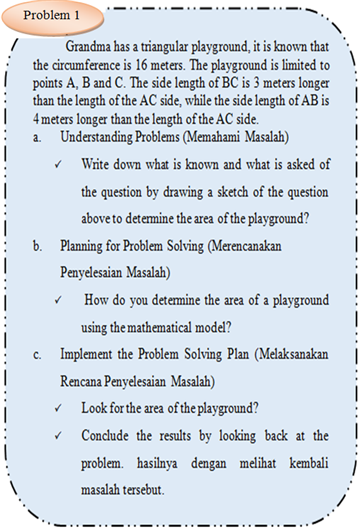• Figure 1. The Problem Solving Sheet Preliminary Tests Mathematical Problem Solving Ability by Students 1
• Figure 2. The Right Answer

The tenth grade students of Public Senior High School 1 Medan answers showed that mathematical problem solving ability level is still low and from the answer sheets showed that the students have not planned yet the right solution based on the problem solving indicator as Figure 1 the wrong answer and Figure 2 the right answer.

From the initial problem analysis concluded that students were still low in understanding the problem, planning problem strategy, solving problem, unable to connect triangle concept and student mathematical understanding was still low. Student mathematical problem solving ability in Public Senior High School 1 Medan was very low. From the initial study test result of mathematical problem solving ability given to 25 students, the gained mean score of student is 40.63 which means based on government regulations on education and culture number 23 of 2016 chapter VI concerning about assessment procedure for article 12 paragraph 2c that student mathematical problem solving ability of Public Senior High School 1 Medan is still in low category” 10.

The low of student mathematical problem solving ability is caused by teacher oriented learning, applying direct learning as learning approach and the teacher’s problem in applying 2013 curriculum which demands the teacher to be creative in developing students thinking about problem solving question. Direct learning strategy is still unable to guide and develop student learning ability, students tend to be passive student and make them unable to understand problem solving question. Juliana and Surya declared “mathematic teaching and learning process will be useful if the learning material given to the student can be understood” 11. The teachers need the right process and the right way to make the students understand the learning, one of that is create effective learning. According to Yuniara and Surya that “the selection of learning strategy and select learning model is one of solution to achieve successful learning” 12.

The National Research Council Mathematic (in Karnasih) expressed “the successful of mathematical problem solving process depends on problem solving representation of the problem” 13. Besides, Fyle and Jhonson expressed “result suggest that minimal computer-generated feedback can be a powerful form of guidance during problem solving” 14. In 2013 curriculum application on math learning emphasizes on modern pedagogic dimension of learning namely using scientific approach involves observing, asking, understanding, attempting, forming networks for all lessons based on government regulation on education and culture of republic of Indonesia number 65 of 2013. Besides, based on government regulation on education and culture of republic of Indonesia number 81 A of 2013 in curriculum of 2013 the students are motivated to check new information with the existing knowledge of student in their mind 15. One of solution to achieve learning is by applying scientific approach. According to Daryanto that “scientific approach is aimed to give understanding to the student in knowing, understanding various material using scientific approach. The information comes from whenever and wherever, it doesn’t depend on one way information namely from the teacher. Therefore, the expected learning conditions to encourage students finding various information is through observation not only told by the teacher” 16. Besides, M. Sudirman, Fatimah & Jupri study result was the scientific learning through quantum learning strategy which applied at the eleventh grade of Senior High School 14 Bandung on math learning can optimize student learning ability so that the students can shape their problem solving ability and self-regulated leaning, gaining optimal mathematic result study and achieving required learning purpose” 8.

As established in the principle of school mathematics learning by NCTM namely “technology is essential in teaching and learning mathematics; it influences the mathematics that is taught and enhances students’ learning” 3. The same explanation on government regulation on national education number 65 that “the using of information technology and communication can improve learning efficient and effectivity” 15. Mathematic learning with computer technology base is essential if it supports with mathematic software. It helps student in doing and analyzing the existing problem. A teacher is required to be able to adjust the software used with the material being taught. One kind of the software is Autograph. According to Karnasih that “autograph is dynamic and multifunction software in mathematic teaching and learning process for junior high school which was developed by Douglas Butler” 13. This software was designed to help students and teachers to visualize mathematic by using three modes namely (1) opportunity and statistic dimension (2) graphic coordinate, transformation, and bivariate data dimension (3) three dimensional space as Figure 3 following.

• Figure 3. Autograph Logo

Karnasih and Sinaga study concluded that “cooperative learning by using dynamic software Autograph in teaching statistic at eleventh grade class Medan improved student problem solving ability. It is showed from the test result that there is significant improvement in problem solving ability. 91.2 percent student liked learning through dynamic software and mathematics teacher is expected to consider the using dynamic software autograph in teaching and learning secondary mathematic especially in statistic learning and other mathematic learning such as algebra, geometric, trigonometry and calculus 17.

Autograph is very potential to help student in one problem solving question as one problem on discovering absolute value concept material.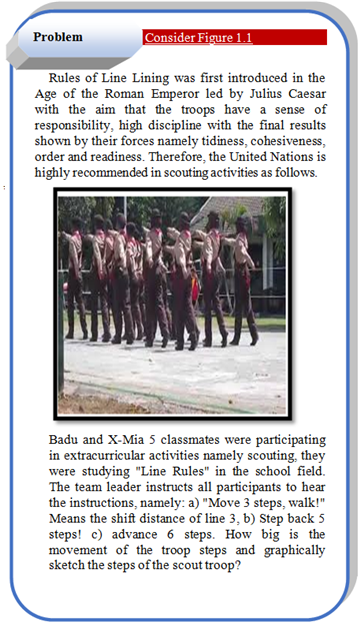The using of software autograph can help students’ understanding and also add students’ knowledge in finishing the problem above as Figure 4 following:

• Figure 4. The Solution to Troubleshooting 1 with Autograph Software Help

By using software Autograph on equality and inequality of linear absolute value one variable material, the students described each graphic of equality and inequality of linear absolute one variable need faster and effective time. The students only need decide the concept of equality and inequality systematically and get the desired description assisted by Autograph. So, students’ opportunity to develop and explore the ability is bigger. It is expected to be able to give effect on building basic concept where it will improve student mathematical problem solving ability and study independence.

Based on the explanations above the writer encourage to conduct a study entitled The Difference of Mathematical Problem Solving Ability Through The Scientific Approach and The Scientific Approach Assisted by Software Autograph.

### 2. Research Methods

This study is quasi experimental study. According to Sugiono “this study uses quasi experimental method with randomized pre-test post-test control group design” 18. The objectives of this study is to analyze whether or not difference between mathematical problem solving ability taught by scientific approach and taught by scientific approach assisted by software Autograph.

2.1. The Instrument and Technique of Collecting Data

To get the complete, right, accurate data and information about things will be examined in this study, the writer chooses the instrument of this study namely mathematical problem solving ability test.

2.1.1. The Initial Mathematical Ability Test (IMAT)

Mathematical initial ability test is aimed to know whether the student mathematical ability in low, medium, or high category before the treatment is given and to see whether or not there is the difference of mathematical initial ability.

On Figure 5 about mathematical initial ability quality mean score diagram (high, medium, low) based on experiment class I and II shows the category of mathematical initial ability (high, medium, low) of each experiment class is not on the same level.

• Figure 5. Average IMAT Score Diagram (High (Tinggi), Medium (Sedang), Low (Rendah))

2.1.2. The Mathematical Problem Solving Ability Test

The test is used to measure student mathematical problem solving ability. The test was given after teaching and learning process with aims to see the difference in student mathematical representation ability after giving treatment. The test instrument of mathematical representation ability was developed from learning material about linear equality and inequalities of one variable absolute values.

2.2. Research Hypothesis Testing Techniques

According Sudjana say that “before the research hypothesis test is carried out, it must first fulfill two conditions, namely the sample of the study is normally distributed and homogeneous. Based on statistical theory The test of hypothesis testing uses two-way variant analysis techniques with a 2x3 factorial design” 19. In this study using two factors, namely: 1) Learning approach consisting of two levels (scientific, scientific assisted software Autograph) and 2) initial mathematical abilities consisting of three levels (High, Medium and Low), so that in the study required 6 combinations different treatments as in the following Table 1 below.

Base Teory Sudjana Statistic Teory 20 the statistical models of this research experiment are as follows: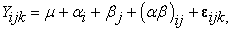(1)

where i = 1,2,3; j = 1,2; k = 1,2,...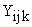: The first student's data (values) on the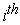first mathematical initial ability and the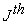learning model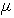: average of all data (large mean, grand mean).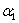: effect of thefirst mathematical initial ability on problem solving abilities.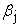: the effect of thelearning approach on problem solving abilities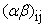: a combination of the effects of thefirst mathematical initial ability and thelearning approach to problem solving abilities.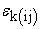: Yijk data deviation of the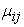population average which is normally distributed with an average of 0.

Arief stated that" the rationale of ANOVA technique is the total variation of all subjects in an experiment can be analyzed into two sources, namely variance between groups and variance in groups. Anova can be used to test two mean or more” 21. According to Kadir that "Two Ways Analysis of Variance is a variance analysis that is influenced by two independent variables on non-independent variables. This variance analysis technique is possible to expand in situations where the things being measured are influenced by two or more variables ” 22. Following are the steps to test the statistical hypothesis by using two-way Anova made by Kadir such us:

a. Calculating the Number of Squares (JK) for several sources of variance, namely Total (T), learning factors denoted by Inter (A), IMAT factors represented by Inter (B), interactions between learning and IMAT are represented by Interaction (AB), and In (D), with the following formula: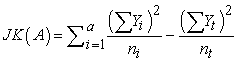(2)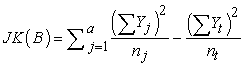(3)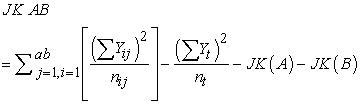(4)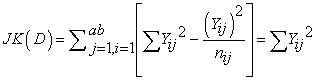(5)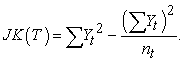(6)

b. Determine the degree of freedom (db) of each source of variance

db (A) = na – 1

db (B) = nb – 1

db (AB) = (na – 1) (nb – 1)

db (D) = nt – (na) (nb)

db (T) = nt – 1.

c. Determine the Average Number of Squares (RJK)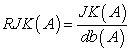(7)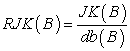(8)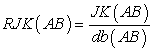(9)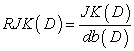(10)

d. Determine F0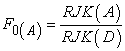(11)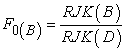(12)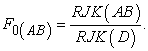(13)

e. Arrange table Anova like Table 2 below:

Test criteria, if F0 > Ftabel at significant level chosen with db numerator is the appropriate db, then H0 is rejected so it is concluded that there are differences in the average increase between the groups tested, on the contrary for F0 ≤ Ftabel, then H0 is accepted. For two-way Anova, the first step is to test the statistical hypothesis of the effect of the interaction, namely F0 (AB). If F0 (AB) ≤ Ftabel or H0 is received, it means that there is no interaction effect, then the next hypothesis is the main effect, namely the F0 (A) test to learn the difference between the average A. Instead, if F0 (AB) > Ftabel or H0 is rejected, meaning there is a significant interaction effect, so the consequence must be a simple effect test. Simple effect is the difference between A in each group Bi = (i = 1, 2, 3).

### 3. Result and Discussion

3.1. Result of Study
3.1.1 Normality and Homogeneity

The summary result of normality test calculation of student mathematical problem solving ability post test data presented on Table 3.

After the treatment given to both of class experiment, based on Table 3 above can be seen that continually significance score is 0.135 for experiment 1 class and 0.144 for experiment 2 class. The significance score of both classes is higher than significance level 0.005 which means H0 which stated that the data was normal for experiment I and experiment II class is accepted. In other words, post-test data for experiment I class which taught by scientific approach assisted by software Autograph and for experiment II class which taught by scientific approach was in normal distribution data. The homogeneity data calculation test of mathematical representation ability is presented on Table 4 following:

From Table 4 was seen that the significance score is 0.969 higher than significance level 0.05 means that H0 which stated that there is no difference of variance between data group is accepted. It shows that both group of post-test data for experiment I class which taught by scientific approach assisted by software Autograph and experiment II class which taught by scientific approach have homogen data variance.

3.2. Test Hypothesis

The post test data came from the normal distribution using Chi Squares, that is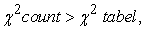then H0 rejected the reject criteria H0 if sig <0.05 the reject criteria H0 if sig <0.05. For experimental class I (0.135)> 0.05, while for experimental class II (0.144)> 0.05. Furthermore, the population variance of the two groups was homogeneous with the accept criteria H0 if sig> 0.05, with the acquisition of 0.969> 0.05. The test results show that the sample comes from a population that is normally distributed with the variance of each pair of homogeneous data groups, then the two-way Anova statistical analysis is then carried out. The following is the formula for the statistical hypothesis:

Hypothesis 1:

H0: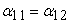Ha: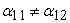Information: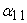= The average mathematical problem solving ability of students with a scientific learning approach assisted by Autograph software.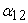= The average mathematical problem solving ability of students with the scientific learning approach.

Hypothesis 2:

H0: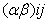= 0

Ha: Minimum one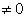Information: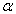: The average initial ability group is mathematical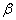: Average learning approach

i = 1,2 dan j = 1,2,3

The testing criteria is If Fcount > Ftabel,, H0 is rejected

The results of the calculation of the two-ways ANOVA test mathematical problem solving abilities of students with the help of Microsoft Excel are presented in Table 5 as follows.

In Table 5 above shows the results of testing hypothesis 1 and Hypothesis 2 with the testing criteria If Ha:then Ha is accepted. The results of the Hypothesis 1 test are obtained F (A) count ≥ F (A) table = 6.150 ≥ 3.9840 then Ho is rejected by the conclusion that at the 95% confidence level there is a difference between mathematical problem solving abilities of students who obtain the scientific assisted Autograph software learning approach with students who obtained the scientific learning approach with a difference of = 0. 0704.

The F(B) count ≥ F(B) table = 23.882 ≥ 3.1338 then Ho is rejected with the conclusion that at the 95% level of confidence there is a difference between the students' mathematical problem solving abilities who obtain the scientific assisted Autograph software learning approach with students with high IMAT, IMAT is and IMAT is low with a difference of 0.4023. Hypothesis 2 test results with Ho reject criteria are F (A x B) count <F (A x B) table = 3.119 < 3.1338 then Ho is accepted by the conclusion that at the 95% level of confidence there is no interaction model learning approach factor and factor IMAT on mathematical problem solving ability with a difference of 0.0587.

The first and the second hypothesis test result by ANOVA two way test used SPSS program was described in Table 6 following:

Based on Table 6 was seen that:

a. Value F from Learning Approach = 0.215 with p-value = 0.049 <0.05 then Ho is rejected and Ha is accepted. This means that there are differences in the average learning independence between students taught by the scientific learning approach assisted by software Autograph and students taught by scientific approach.

b. For IMAT = 116,033 with p-value = 0.00 <0.05 then Ho is rejected and Ha is accepted. This means that there are differences in the average learning independence of students between students who have high, medium and low initial abilities.

c. Interaction between Learning Approaches * IMAT = 0.38 with p-value = 0.985> 0.05 then Ho is rejected and Ha is accepted. This means that there is no interaction between the learning approach and IMAT on student learning independence. It shows that there is difference of student mathematical problem solving ability taught by scientific approach assisted by software autograph and taught by scientific approach. It means H0 is rejected and Ha is accepted. It shows that there is interaction between learning approach (scientific approach and scientific approach assisted by software Autograph) and students IMAT towards student mathematical solving ability. It also can be described on Figure 6.

From Figure 6, the mean score of student mathematical solving ability is low in IMAT category on scientific approach assisted by software autograph, higher than low IMAT category in the assisted scientific approach class. Meanwhile, on IMAT category whether low, medium and high the mean score shows that there is no interaction between learning approach (scientific approach assisted by software Autograph and scientific approach) and student IMAT towards student mathematical problem solving ability. It means that there is effect of learning approach which contributed to improve students toward students mathematical problem solving ability.

• Figure 6. Graph of Interaction between Learning Approach and KAM against Students' Mathematical Solving Ability

### 4. Conclusion

Based on study data result analysis of student mathematical problem solving ability taught by scientific approach assisted by software autograph, here are some conclusions:

1. There is difference of student mathematical problem solving ability taught by scientific approach assisted by software Autograph and scientific approach

2. There is no interaction between learning approach (scientific approach assisted by software Autograph and scientific approach) and IMAT towards student mathematical problem solving abiity. It means that there is effect of learning approach which contributed to improve students toward student mathematical problem solving ability.

### 5. Suggestion

Based on conclusion mentioned above, the writer would like to deliver some suggestions, they are:

1. Teacher needs to give more understanding to student in understanding the question and representing mathematical problem especially on indicator of mathematical problem solving plan

2. Apply scientific approach assisted by software Autograph as alternative approach to maximize student mathematical problem solving ability

3. The related institution should held socialization, training, and model development or learning approach especially about scientific approach assisted by software Autograph so it can be well-known by all teacher and education staff in improving student mathematical solving ability.

### References

  Napitupulu, E. E, & Abil, M. 2008. Kemampuan Pemecahan Masalah Matematis Siswa (Studi Kasus di SMA Negeri parompong Kabupaten Bandung Barat). Jurnal pendidikan Matematika PARADIKMA. (Online), Vol. 1 Number 1, Page 24-33. In article  Soriyah, K. Edy S. & Edy, S. 2017. Membangun Kemampuan Pemecahan Masalah dan Kemandirian Belajar Siswa Melalui Pembelajaran Geometri Berbasis Pendidikan Matematika Realistik. 1-2. In article  National Council of Teacher of Mathematics. 2000. Principles and Standards for School Mathematics. Reston. VA: NCTM. Page 52. In article  Polya, G. 1973. How To Solve It, Second Edition. New Jersey: Princeton University Press. Page 52. In article  Szetela, W. & Nicol, W. 1982. Evaluating Problem Solving in Mathematics. New York: Cambridge University Press. Page 2. In article  Schoenfeld, A.H. (1992). Learning To Think Mathematically: Problem Solving, Metacognition, And Sense Making İn Mathematics. In D.A. Grouws (Ed.), Handbook of Research on Mathematics Teaching and Learning New York: Macmillan. Page 334-370. In article  Rahmawati. 2016. Result Presentation TIMSS 2015. Page 3. In article  M. Sudirman. S. Fatimah. & A. Jupri. 2017. Improving Problem Solving Skill and Self Regulated Learning of Senior High School Students through Scientific Approach using Quantum Learning strategy. International Journal of Science and Applied Science. (Online). Vol. 2, No. 1 (2017). Page 251, Page 254. In article  Napitupulu, E. Suryadi, D. & Kusumah, Y.S. 2016. Cultivating Ultivating Upper Secondary Students’ Mathematical Reasoning-Ability and Attitude Towards Mathematics through Problem-Based Learning. Journal on Mathematics Education. (Online), Volume 7, Number 2. Page 124. In article  Permendikbud. 2013. Nomor 23 Tahun 2016 Tentang Standar Penilaian Pendidikan. Jakarta: Menteri Pendidikan dan Kebudayaan Republik Indonesia. In article  Juliana, M., dan Surya, E. 2017. An Analysis Of Jingsaw Cooperative Effectiveness To Improve The Self-Confidance And Learning Result Of Vocation High School Student’s. IJARIIE. Vol. 3-Issue-2. Page. In article  Yuniara, P., Surya, E. 2017. Application of Problem Based Learning to Students’ Improving on Mathematics Concept of Ability. IJSBAR. Vol. 3, No. 33, page 261-269. In article  Kurniasih, I. 2014. Implementasi Kurikulum 2013: teori dan Praktek. Surabaya: Kata Pena.Kemendikbud. 2013. Page 45. In article  Fyfe, E. R & , B. R. 2016. The benefits of computer-generated feedback for mathematics problem solving. (Elselvier). , Pages 140-151. In article View Article  PubMed  Peraturan Menteri Pendidikan Nasional Republik Indonesia Nomor 65 Tahun 2013 Tentang Standar Isi untuk Satuan Pendidikan Dasar dan Menengah. Jakarta: Depdiknas. Page 7. In article  Daryanto.2014. Pendekatan Pembelajaran Saintifik Kurikulum 2013. Yoyakarta: Penerbit Gava Media. Page 51. In article  Karnasih, I. & Sinaga, M. 2014. Enhancing Mathematical Problem Solving and Mathematical Connection Through the Use of Dynamic Software Autograph in Cooperative Learning Think-Pair-Share. SAINSAB. (Online). Vol. 2 No. 1. In article  Sugiyono. 2014. Metode Penelitian Pendidikan (Pendekatan Kuantatif, Kualitatif, dan R& D). Bandung: IKAPI. Page 165. In article  Sudjana. 1989. Desain Dan Analisis Eksperimen Bandung: PT. Tarsito. Page 215. In article  Sudjana. 2003. Teknik Analisis Regresi dan korelasi bagi Para Peneliti. Bandung: PT. Tarsito. Page 113. In article  Arief, F. 2005. Pengantar Penelitian dalam Pendidikan, Cet. II. Yogyakarta: Pustaka Pelajar. In article PubMed  Kadir. 2015. Statistika Terapan. Jakarta: Raja Grafindo Persada. Page 346-347. In article

Published with license by Science and Education Publishing, Copyright © 2018 Rismalyah Manalu, E.Elvis Napitupulu, Martua Manullang, Delima Simanjuntak and Jetti H. SinambelaThis work is licensed under a Creative Commons Attribution 4.0 International License. To view a copy of this license, visit http://creativecommons.org/licenses/by/4.0/